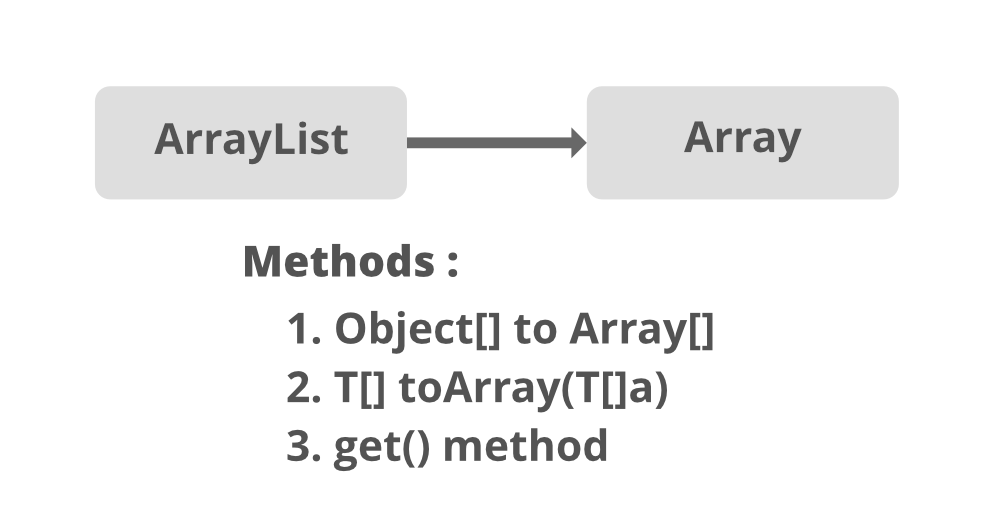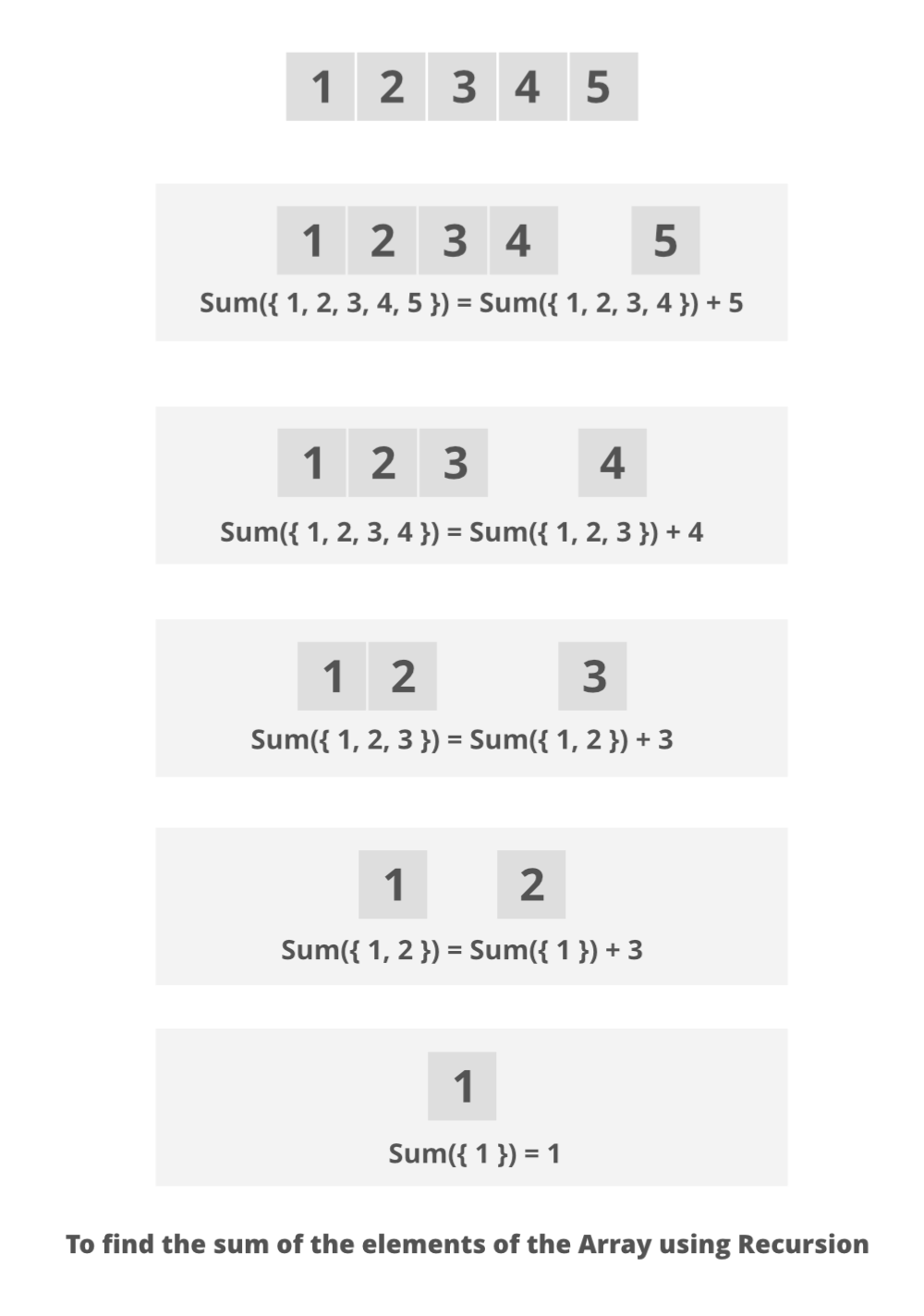# Java Program to Compute the Sum of Numbers in a List Using Recursion

• Difficulty Level : Medium
• Last Updated : 26 May, 2022

ArrayList is a part of the Collection framework and is present in java.util package. It provides us with dynamic arrays in Java. Though, it may be slower than standard arrays but can be helpful in programs where lots of manipulation in the array is needed. This class is found in java.util package.

Illustration:

```Input  : [1, 3, 9]
Output : 13```

Here the naive method can be to add up elements of List and maintain a counter in which sum is stored while traversing List. The step ahead method can be to convert the List to the array and do the same. Now more optimal method can be to use recursion while doing so in which subpart of List or array is automatically computed by recursion principles. Here this optimal approach is described and implemented as shown.

Methods:

1. Converting ArrayList to arrays and using recursion principles over arrays.

Method 1: Converting ArrayList to arrays and using recursion principles over arrays.It is achieved by converting ArrayList to arrays and using recursion principles over arrays. Recursion in the list to array conversion and computing sum of elements using add() method.

Approach:

1. Take the elements of the list as input from the user.
2. Convert the list into an array of the same size.
3. Add the elements to it.
4. Compute the sum of arrays using recursion principles.Example

## Java

 `// Java Program to Compute Sum of Numbers in a List` `// by converting to arrays and applying recursion`   `// Importing java input/output classes` `import` `java.io.*;` `// Importing List and ArrayList class from` `// java.util package` `import` `java.util.ArrayList;` `import` `java.util.List;`   `// Class` `public` `class` `GFG {`   `    ``// Method to calculate sum recursively` `    ``public` `static` `int` `sumOfArray(Integer[] a, ``int` `n)` `    ``{` `        ``if` `(n == ``0``)` `            ``return` `a[n];` `        ``else` `            ``return` `a[n] + sumOfArray(a, n - ``1``);` `    ``}`   `    ``// Method- main()` `    ``public` `static` `void` `main(String[] args)` `    ``{` `        ``// Creating a List of Integer type` `        ``// Declaring an object- 'al'` `        ``List al = ``new` `ArrayList();`   `        ``// Adding elements to the List` `        ``// Custom inputs` `        ``al.add(``1``);` `        ``al.add(``2``);` `        ``al.add(``3``);` `        ``al.add(``4``);` `        ``al.add(``5``);`   `        ``// Converting above List to array` `        ``// using toArray() method` `        ``Integer a[] = ``new` `Integer[al.size()];` `        ``al.toArray(a);`   `        ``// Display message` `        ``System.out.print(``"Elements in List : "``);`   `        ``// Printing array of objects` `        ``// using for each loop` `        ``for` `(Integer obj : a) {` `            ``System.out.print(obj + ``" "``);` `        ``}`   `        ``// Recursion math to calculate sum snd` `        ``// storing sum in a variable` `        ``int` `sum = sumOfArray(a, a.length - ``1``);`   `        ``// Next line` `        ``System.out.println();`   `        ``// Print the sum returned above` `        ``System.out.println(``"Sum of elements  : "` `+ sum);` `    ``}` `}`

Output

```Elements in List : 1 2 3 4 5
Sum of elements  : 15```

This method appends the specified element to the end of this list

Syntax:

`public boolean add(E element) ;`

Parameter: Object to be appended to this list.

Return Type: It will always return a boolean true and the signature is as so because other classes in collections family need a return type.

Exceptions: NA

Example:

## Java

 `// Java Program to Compute the Sum of Numbers in a List ` `// using Recursion via ArrayList.add() method`   `// Importing all classes of ` `// java.util package` `import` `java.util.*;`   `// Class` `public` `class` `GFG` `{   ` `  ``// Declaring variables outside main class` `  ``int` `sum = ``0``, j = ``0``;`   `  ``// Main driver method` `  ``public` `static` `void` `main(String[]args)` `  ``{ ` `    ``/*` `    ``// Taking the input from the user ` `    ``int n;` `    ``Scanner s = new Scanner(System.in);`   `    ``// Display message ` `    ``System.out.print("Enter the no. of elements :");` `    `  `    ``// Reading integer elements using nextInt() method` `    ``n = s.nextInt();`   `    ``// Display message ` `    ``System.out.println("Enter all the elements you want:");` `    ``*/`   `    ``// Creating an object of List of Integer type ` `    ``List < Integer > list = ``new` `ArrayList < Integer > ();`   `    ``// Adding elements to object of List` `    ``// Custom inputs to show sum` `    ``list.add(``10``);` `    ``list.add(``90``);` `    ``list.add(``30``);` `    ``list.add(``40``);` `    ``list.add(``70``);` `    ``list.add(``100``);` `    ``list.add(``0``);` `    `  `    ``System.out.println(``"Elements in List : "` `+ list);` `    ``/*` `    ``// If input is through user than` `    `  `    ``// For loop to add elements inside List` `    ``for (int i = 0; i < n; i++)` `    ``{` `      ``// Adding integer elements in the list` `      ``list.add(s.nextInt()); ` `    ``}` `    ``*/`   `    ``// Converting List to Array` `    ``Integer[] a = list.toArray(``new` `Integer[list.size()]);`   `    ``// Initialising object of Main class` `    ``GFG elem = ``new` `GFG();`   `    ``// Finding sum of elements in array ` `    ``// via add() method using recursion` `    ``int` `x = elem.add(a, a.length, ``0``);` `    `  `    ``// Print the sum of array/elements initially in List` `    ``System.out.println(``"Sum of elements in List :"` `+ x);` `  ``}`   `  ``// add() method to add elements in array` `  ``// using recursion` `  ``int` `add(Integer arr[], ``int` `n, ``int` `i)` `  ``{` `    ``if``(i < n)` `    ``{` `        ``return` `arr[i] + add(arr, n, ++i);` `    ``}` `    ``else` `    ``{` `        ``return` `0``;` `    ``}` `  ``}` `}`

Output

```Elements in List : [10, 90, 30, 40, 70, 100, 0]
Sum of elements in List :340```

My Personal Notes arrow_drop_up
Recommended Articles
Page :